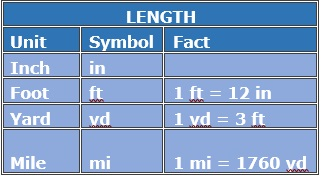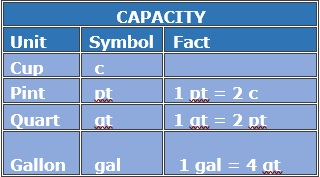# U.S. Customary Unit Conversion with Whole Number Values1 mile = 1,760 yards = 5,280 feet = 63,360 inches1 gallons = 4 quarts = 8 pints = 16 cups1 ton = 2000 pounds = 32000 ounces

Convert between yards (yd) and feet (in)

4 yd = __feet

### Solution

Step 1:

3 ft = 1 yd

Step 2:

So, 4 yd = 4 x 3 ft = 12 ft

Convert between ounces (oz) and pounds (lb).

32 oz = __lb

### Solution

Step 1:

1 lb = 16 oz; 1 oz = $\frac{1}{16}$ lb

Step 2:

So, 32 oz = $\frac{32}{16}$ lb = 2 lb

Convert between quarts(qt) and gallons(gal)

20 qt = __gal

### Solution

Step 1:

1 gal = 4 qt; 1 qt = $\frac{1}{4}$ gal

Step 2:

So, 20 qt = $\frac{20}{4}$ gal = 5 gal